11. Let R and S be two fuzzy relations defined as follows. Then, the resulting relation, T, which relates elements of universe of X to elements of universe of Z using max-product composition is given by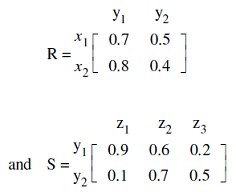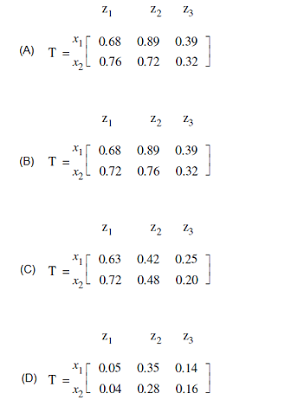a. A b. B c. C d. D

 12. Let R and S be two fuzzy relations defined as follows. Then, the resulting relation, T, which relates elements of universe x to elements of universe z using max-min composition is given by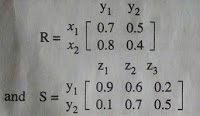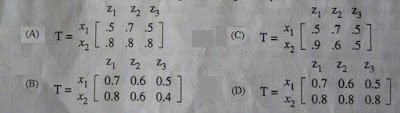a. A b. B c. C d. D

 13. Compute the value of adding the following two fuzzy integers:A = {(0.3,1), (0.6,2), (1,3), (0.7,4), (0.2,5)}B = {(0.5,11), (1,12), (0.5,13)}Where fuzzy addition is defined asμA+B(z) = maxx+y=z (min(μA(x), μB(x)))Then, f(A+B) is equal to a. {(0.5,12), (0.6,13), (1,14), (0.7,15), (0.7,16), (1,17), (1,18)} b. {(0.5,12), (0.6,13), (1,14), (1,15), (1,16), (1,17), (1,18)} c. {(0.3,12), (0.5,13), (0.5,14), (1,15), (0.7,16), (0.5,17), (0.2,18)} d. {(0.3,12), (0.5,13), (0.6,14), (1,15), (0.7,16), (0.5,17), (0.2,18)}
 Answer: (d).{(0.3,12), (0.5,13), (0.6,14), (1,15), (0.7,16), (0.5,17), (0.2,18)}

 14. A perceptron has input weights W1 = -3.9 and W2 = 1.1 with threshold value T = 0.3. What output does it give for the input x1 = 1.3 and x2 = 2.2? a. -2.65 b. -2.30 c. 0 d. 1

 15. Let A and B be two fuzzy integers defined as:A={(1,0.3), (2,0.6), (3,1), (4,0.7), (5,0.2)}B={(10,0.5), (11,1), (12,0.5)}Using fuzzy arithmetic operation given by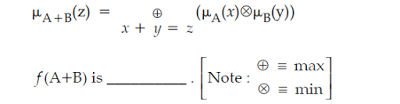a. {(11,0.8), (13,1), (15,1)} b. {(11,0.3), (12,0.5), (13,1), (14,1), (15,1), (16,0.5), (17,0.2)} c. {(11,0.3), (12,0.5), (13,0.6), (14,1), (15,1), (16,0.5), (17,0.2)} d. {(11,0.3), (12,0.5), (13,0.6), (14,1), (15,0.7), (16,0.5), (17,0.2)}
 Answer: (d).{(11,0.3), (12,0.5), (13,0.6), (14,1), (15,0.7), (16,0.5), (17,0.2)}

 16. Let A be the set of comfortable houses given as follows. Then the set of comfortable and affordable houses is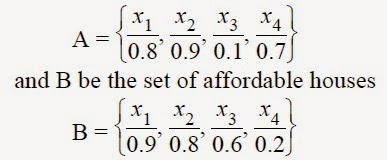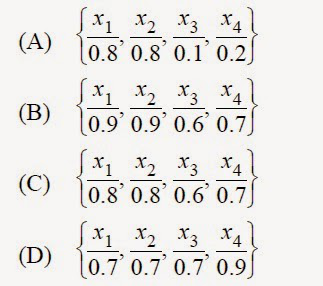a. A b. B c. C d. D

 17. Support of a fuzzy set given below, within a universal set X is given as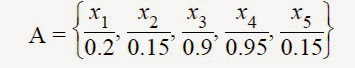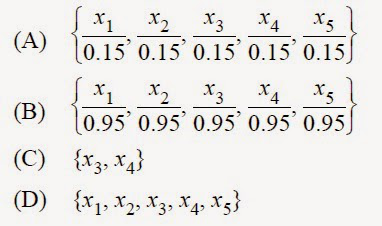a. A b. B c. C d. D